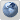Telcobridges - Session Border Controllers
Would you like to react to this message? Create an account in a few clicks or log in to continue.

# Mean Opinion Score (MOS) Support##Mean Opinion Score (MOS) Support

Toolpack uses a well-known model to evaluate a MOS value based on collected statistics for a call. There are 3 factors that significantly impact the call quality: latency, packet loss and jitter of RTP packets. Using these statistics, we calculate an R-value that ranges from 1 to 100 where a higher number is better. Finally the MOS is calculated using this formula.

MOS = 1 + 0.035R + ((R - 60) * (100 – R) * 0.000007R)

For the MOS calculated from the R-value, there is no notion of codec. We decided to apply a ratio in relation to the codec's ideal MOS.

See the table below:Network quality
TelcoBridges' MOS implementation adds another concept over the standard MOS: Network quality. The network quality parameter provides a fast way to identify a bad network condition. The MOS is useful when comparing calls for the same NAP or for the same network. But if you are using several codecs, the MOS value might be misleading to detect network problems.

• Examples

• A perfect network (i.e. no packet loss, no latency) using G.723 will have a MOS of 3.6. This is relatively low, but it represents a network quality of 100%. The only way to improve the MOS will be to use a higher quality codec.

• On the other hand, a G.711 call with a MOS of 3.7 represents a network quality of 86%. This rather low and based on these values, you know there is something that can be improved in the network.

Ingress-EgressThere are 2 MOS values calculated per call leg. The ingress value is using RTP reception statistics and the egress value is using RTCP statistics received from the peer. Using these statistics, w can calculate MOS and network quality for the ingress and egress directions.When using Call Trace, the lowest value between ingress and egress MOS and network quality are displayed.[/list]